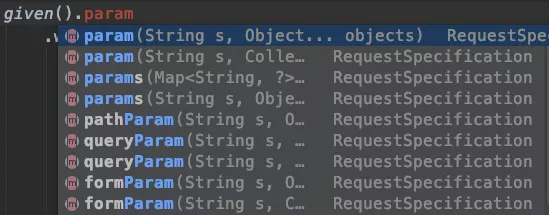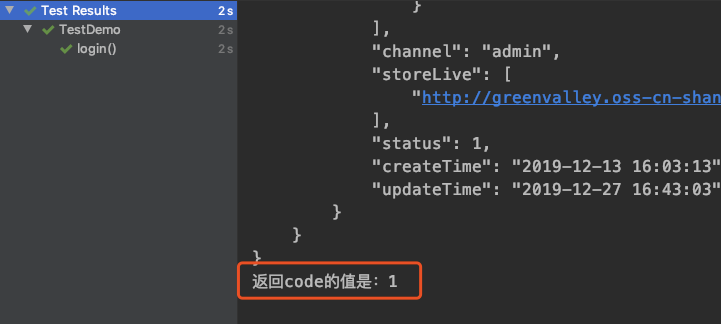# 利器 | Java 接口自动化测试首选方案：REST Assured 实践 (一)1 . 初识 REST Assured

1.1 优点：

REST Assured 官方的 README 第一句话对进行了一个优点的概述，总的意思表达的就是简单好用。那么 REST Assured 有哪些优点，又该如何使用呢？

• 开源
• 简约的接口测试 DSL
• 支持 `xml` `json ` 的结构化解析
• 支持 `xpath` `jsonpath` `gpath ` 等多种解析方式
• `spring ` 的支持比较全面

2. 如何使用

``````<dependency>
``````
``````   <groupId>io.rest-assured</groupId>
``````
``````    <artifactId>rest-assured</artifactId>
``````
``````    <version>4.0.0</version>
``````
``````    <scope>test</scope>
``````
``````</dependency>
``````

2.1 基本三步曲

``````//使用参数
``````
``````given().
``````
``````    param("key1", "value1").
``````
``````    param("key2", "value2").
``````
``````when().
``````
``````    post("/somewhere").
``````
``````then().
``````
``````    body(containsString("OK"))
``````
``````
``````
``````//使用X-Path (XML only)
``````
``````given().
``````
``````    params("firstName", "John", "lastName", "Doe").
``````
``````when().
``````
``````    post("/greetMe").
``````
``````then().
``````
``````    body(hasXPath("/greeting/firstName[text()='John']"))
``````

2.2 分步拆解

`http://47.103.xxx.133/auth/oauth/token`

``````{
``````
``````  "password": "elcrD28ZSLLtR0VLs/jERA\u003d\u003d\n",
``````
``````  "grant_type": "password",
``````
``````  "scope": "server",
``````
``````  "userType": 1,
``````
``````  "username": "xxx"
``````
``````}
``````

Request Header 如下：

``````Headers:    Authorization=Basic c3lzdGVtxxxRlbQ==
``````
``````        Host=47.103.xxx.133
``````
``````        Accept=*/*
``````
``````        Content-Type=application/json; charset=ISO-8859-1
``````

Givern• param通常我们都会使用 given().param 方法来传参，REST Assured 会根据 HTTP 方法自动尝试确定哪种参数类型（即查询或表单参数），如果是 GET，则查询参数将自动使用，如果使用 POST，则将使用表单参数；

• queryParam 和 formParam有时候在 PUT 或 POST 请求中，需要区分查询参数和表单参数时，就需要使用queryParam 和 formParam 方法了，具体写法如下：

``````given().
``````
``````       formParam("formParamName", "value1").
``````
``````       queryParam("queryParamName", "value2").
``````
``````when().
``````
``````       post("/something")
``````
• pathParam
使用 `given` 时指定请求路径的参数，这个方法很少用到，或者说我本人几乎没用到过(可能我的修行还不够，踩坑还太少~)；具体写法如下：
``````given().
``````
``````        pathParam("OAuth", "oauth").
``````
``````        pathParam("accessToken", "token").
``````
``````when().
``````
``````        post("/auth/{OAuth}/{accessToken}").
``````
``````then().
``````

header/headers

``````given()
``````
``````       .header("Authorization","Basic c3lzdGVtOxxxbQ==")
``````
``````       .header("Host","47.xxx.xxx.133")
``````

``````given()
``````
``````       .headers("Authorization","Basic c3lzdGVtxxx3RlbQ==","Host","47.xxx.xxx.133")
``````

cookie

``````given()
``````
``````      .cookie("c_a","aaaaaa")
``````
``````      .cookie("c_b","bbbbbb"). ..
``````

contentType

``````given().contentType("application/json"). ..
``````
``````//或者
``````
``````given().contentType(ContentType.JSON). ..
``````

body
`POST` , `PUT``DELETE` 请求中，我们经常还需要带上请求体body，写法如下：

``````given().body("{\n" +
``````
``````                "\t\"password\": \"elcrD28xxxR0VLs/jERA\\u003d\\u003d\\n\",\n" +
``````
``````                "\t\"grant_type\": \"password\",\n" +
``````
``````                "\t\"scope\": \"server\",\n" +
``````
``````                "\t\"userType\": 1,\n" +
``````
``````                "\t\"username\": \"xxx\"\n" +
``````
``````                "}")
``````

``````given().request().body("{\n" +
``````
``````                "\t\"password\": \"elcrD28xxxR0VLs/jERA\\u003d\\u003d\\n\",\n" +
``````
``````                "\t\"grant_type\": \"password\",\n" +
``````
``````                "\t\"scope\": \"server\",\n" +
``````
``````                "\t\"userType\": 1,\n" +
``````
``````                "\t\"username\": \"xxx\"\n" +
``````
``````                "}")
``````

get("/lotto").then().assertThat().body(“lotto.lottoId”, equalTo(5));

proxy

given().proxy(“127.0.0.1”,8888). …

When

• `when` 主要用来触发请求，在 `when` 后面接着请求URL：

given().when().post(“http://47.103.xxx.133/auth/oauth/token”). …

``````given()
``````
``````    .when()
``````
``````        .contentType(ContentType.JSON)
``````
``````        .headers("Authorization","Basic c3lzxxx3RlbQ==","Host","47.xxx.xxx.133")
``````
``````        .request().body("{\n" +
``````
``````            "\t\"password\": \"elcrD28ZSLLtR0VLs/jERA\\u003d\\u003d\\n\",\n" +
``````
``````            "\t\"grant_type\": \"password\",\n" +
``````
``````            "\t\"scope\": \"server\",\n" +
``````
``````            "\t\"userType\": 1,\n" +
``````
``````            "\t\"username\": \"qinzhen\"\n" +
``````
``````            "}")
``````
``````        .post("http://47.xxx.xxx.133/auth/oauth/token")
``````

Then

then后面可以跟断言，也可以获取响应值

• 断言-then().body()
then().body() 可以对响应结果进行断言，在 body 中写入断言：
``````.. post("http://47.xxx.xxx.133/auth/oauth/token")
``````
``````   .then().statusCode(200).body("code",equalTo(1));
``````

``````.. .then()
``````
``````        .log().all().statusCode(200).body("code",equalTo(1))
``````
``````        .extract().body().path("code");
``````

``````body("{\n" +
``````
``````    "\t\"password\": \"elcrD28ZxxxVLs/jERA\\u003d\\u003d\\n\",\n" +
``````
``````    "\t\"grant_type\": \"password\",\n" +
``````
``````    "\t\"scope\": \"server\",\n" +
``````
``````    "\t\"userType\": 1,\n" +
``````
``````    "\t\"username\": \"qinzhen\"\n" +
``````
``````    "}")
``````

``````HashMap map = new HashMap();
``````
``````map.put("password","elcrD28ZSLLtR0VLs/jERA\u003d\u003d\n");
``````
``````map.put("grant_type","password");
``````
``````map.put("scope","server");
``````
``````map.put("userType",1);
``````
``````map.put("username","xxx");
``````
``````given()
``````
``````      .headers("Authorization","Basic c3lzdGVtxxxlbQ==","Host","47.xxx.xxx.133")
``````
``````      .contentType(JSON)
``````
``````      .body(map). ..
``````

``````HashMap map = new HashMap();
``````
``````map.put("password","elcrD28ZSLLtR0VLs/jERA\u003d\u003d\n");
``````
``````map.put("grant_type","password");
``````
``````map.put("scope","server");
``````
``````map.put("userType",1);
``````
``````map.put("username","xxx");
``````
``````Integer code =
``````
``````given()
``````
``````    .headers("Authorization","Basic c3lzdGVtxxxlbQ==","Host","47.xxx.xxx.133")
``````
``````    .contentType(JSON)
``````
``````    .body(map).
``````
``````when()
``````
``````    .log().all().post("http://47.xxx.xxx.133/auth/oauth/token").
``````
``````then()
``````
``````    .log().all().statusCode(200).body("code",equalTo(1))
``````
``````    .extract().body().path("code");
``````
``````System.out.println("返回code的值是："+code);
``````